# Introduction

These notes should enable the user to estimate phylogenetic trees from alignment data with different methods using the phangorn package (Schliep 2011) . Several functions of package are also described in more detail in (Paradis 2012). For more theoretical background on all the methods see e.g. (Felsenstein 2004)(Yang 2006). This document illustrates some of the package features to estimate phylogenetic trees using different reconstruction methods. Small adaptations to the scripts in section @ref(appendix) should enable the user to perform phylogenetic analyses.

# Getting started

The first thing we have to do is to read in an alignment. Unfortunately there exists many different file formats that alignments can be stored in. The function read.phyDat is used to read in an alignment. There are several functions to read in alignments depending on the format of the data set (“nexus,” “phylip,” “fasta”) and the kind of data (amino acid or nucleotides) in the ape package (Paradis and Schliep 2019) and phangorn. The function read.phyDat calls these other functions and transform them into a phyDat object. For the specific parameter settings available look in the help files of the function read.dna (for phylip, fasta, clustal format), read.nexus.data for nexus files. For amino acid data additional read.aa is called.

library(ape)
library(phangorn)
fdir <- system.file("extdata/trees", package = "phangorn")
format = "interleaved")

# Distance based methods

After reading in the alignment we can build a first tree with distance based methods. The function dist.dna from the ape package computes distances for many DNA substitution models. To use the function dist.dna we have to transform the data to class DNAbin. For amino acids the function dist.ml offers common substitution models (for example “WAG,” “JTT,” “LG,” “Dayhoff,” “cpREV,” “mtmam,” “mtArt,” “MtZoa” or “mtREV24”).

After constructing a distance matrix we reconstruct a rooted tree with UPGMA and alternatively an unrooted tree using Neighbor Joining (Saitou and Nei 1987)(Studier and Keppler 1988). More distance methods like fastme are available in the ape package.

dm  <- dist.ml(primates)
treeUPGMA  <- upgma(dm)
treeNJ  <- NJ(dm)

We can plot the trees treeUPGMA and treeNJ with the commands:

plot(treeUPGMA, main="UPGMA")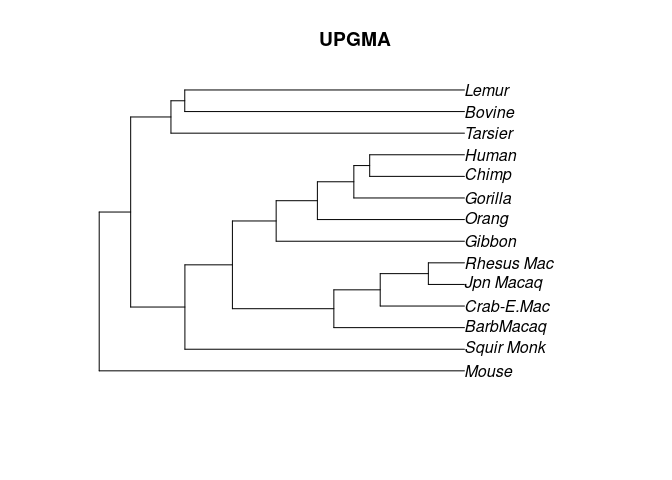Rooted UPGMA tree.

plot(treeNJ, "unrooted", main="NJ")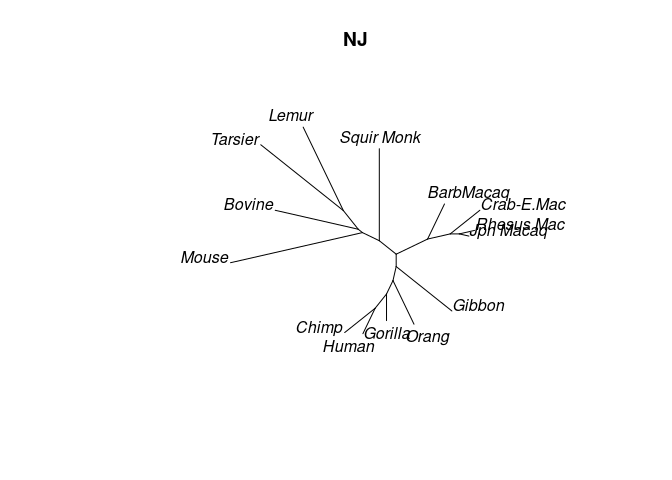Unrooted NJ tree.

## Bootstrap

To run the bootstrap we need to first write a function which computes a tree from an alignment. So we first need to compute a distance matrix and afterwards compute the tree. This function we can than give to the bootstrap.phyDat function.

fun <- function(x) upgma(dist.ml(x))
bs_upgma <- bootstrap.phyDat(primates,  fun)

With the new syntax of R 4.1 this can be written a bit shorter:

bs_upgma <- bootstrap.phyDat(primates,  $$x){dist.ml(x) |> upgma}) Finally we can plot the tree with bootstrap values added: plotBS(treeUPGMA, bs_upgma, main="UPGMA")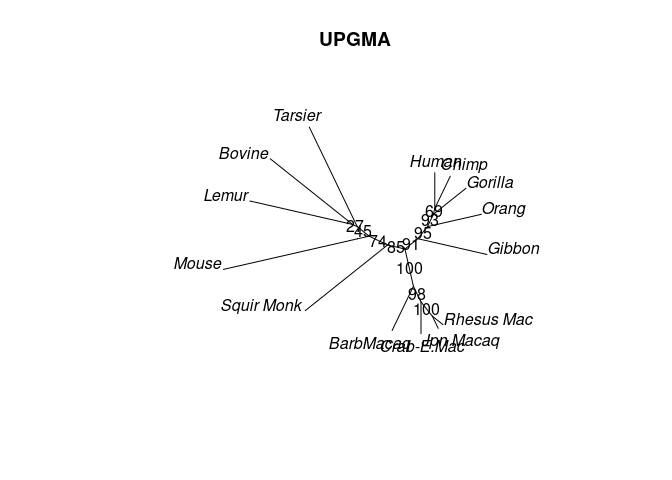Rooted UPGMA tree. Distance based methods are very fast and we will use the UPGMA and NJ tree as starting trees for the maximum parsimony and maximum likelihood analyses. # Parsimony The function parsimony returns the parsimony score, that is the number of changes which are at least necessary to describe the data for a given tree. We can compare the parsimony score or the two trees we computed so far: parsimony(treeUPGMA, primates) ##  751 parsimony(treeNJ, primates) ##  746 The function optim.parsimony performs tree rearrangements to find trees with a lower parsimony score. The tree rearrangement implemented are nearest-neighbor interchanges (NNI) and subtree pruning and regrafting (SPR). The later one only works so far with the fitch algorithm. treePars <- optim.parsimony(treeUPGMA, primates) ## Final p-score 746 after 1 nni operations However is also a version of the parsimony ratchet (Nixon 1999) implemented, which is likely to find better trees than just doing NNI / SPR rearrangements. The current implementation 1. Create a bootstrap data set \(D_b$$ from the original data set.
2. Take the current best tree and perform tree rearrangements on $$D_b$$ and save bootstrap tree as $$T_b$$.
3. Use $$T_b$$ and perform tree rearrangements on the original data set. If this trees has a lower parsimony score than the currently best tree replace it.
4. Iterate 1:3 until either a given number of iteration is reached or no improvements have been recorded for a number of iterations.
treeRatchet  <- pratchet(primates, trace = 0)
parsimony(c(treePars, treeRatchet), primates)
##  746 746

Next we assign branch length to the tree. The branch length are proportional to the number of substitutions / site.

treeRatchet  <- acctran(treeRatchet, primates)

The parsimony ratchet implicitly performs a bootstrap analysis (step 1). We make use of this and store the trees which where visited. This allows us to add bootstrap support values to the tree.

plotBS(midpoint(treeRatchet), type="phylogram")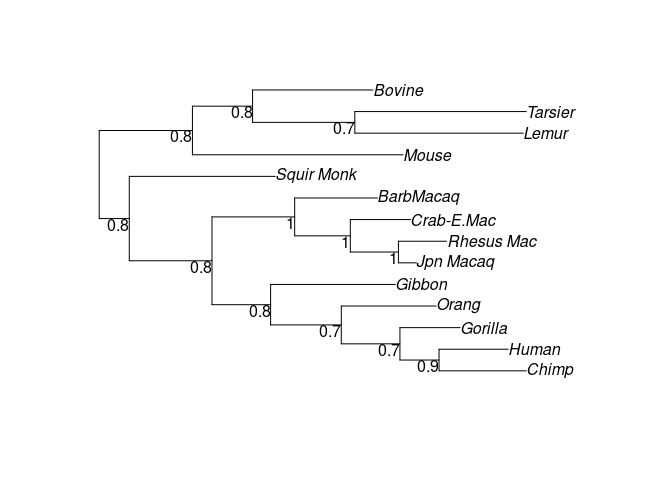## Branch and bound

For data sets with few species it is also possible to find all most parsimonious trees using a branch and bound algorithm (Hendy and Penny 1982). For data sets with more than 10 taxa this can take a long time and depends strongly on how tree like the data are. And for more than 20-30 taxa this will take almost forever.

(trees <- bab(subset(primates,1:10)))
## 1 phylogenetic tree

# Maximum likelihood

The last method we will describe in this vignette is Maximum Likelihood (ML) as introduced by Felsenstein (Felsenstein 1981). We can easily compute the likelihood for a tree given the data

fit = pml(treeNJ, data=primates)
fit
##
##  loglikelihood: -3075
##
## unconstrained loglikelihood: -1230
##
## Rate matrix:
##   a c g t
## a 0 1 1 1
## c 1 0 1 1
## g 1 1 0 1
## t 1 1 1 0
##
## Base frequencies:
## 0.25 0.25 0.25 0.25

The function pml returns an object of class pml. This object contains the data, the tree and many different parameters of the model like the likelihood. There are many generic functions for the class pml available, which allow the handling of these objects.

methods(class="pml")
##  AICc   BIC    anova  logLik plot   print  simSeq update vcov
## see '?methods' for accessing help and source code

The object fit just estimated the likelihood for the tree it got supplied, but the branch length are not optimized for the Jukes-Cantor model yet, which can be done with the function optim.pml.

fitJC  <- optim.pml(fit, TRUE)
## optimize edge weights:  -3075 --> -3068
## optimize edge weights:  -3068 --> -3068
## optimize topology:  -3068 --> -3068
## optimize topology:  -3068 --> -3068
## 1
## optimize edge weights:  -3068 --> -3068
## optimize topology:  -3068 --> -3068
## 0
## optimize edge weights:  -3068 --> -3068
logLik(fitJC)
## 'log Lik.' -3068 (df=25)

With the default valuespml will estimate a Jukes-Cantor model. The generic function update allows to change parameters. We will change the model to the GTR + $$\Gamma(4)$$ + I model and then optimize all the parameters.

fitGTR <- update(fit, k=4, inv=0.2)
fitGTR <- optim.pml(fitGTR, model="GTR", optInv=TRUE, optGamma=TRUE,
rearrangement = "NNI", control = pml.control(trace = 0))
fitGTR
##
##  loglikelihood: -2614
##
## unconstrained loglikelihood: -1230
## Proportion of invariant sites: 0.007799
## Discrete gamma model
## Number of rate categories: 4
## Shape parameter: 3.946
##
## Rate matrix:
##         a       c        g      t
## a  0.0000 0.50805 18.75080 0.3147
## c  0.5081 0.00000  0.01293 8.2089
## g 18.7508 0.01293  0.00000 1.0000
## t  0.3147 8.20888  1.00000 0.0000
##
## Base frequencies:
## 0.3844 0.3823 0.04015 0.1932

With the control parameters the thresholds for the fitting process can be changed. Here we want just to suppress output during the fitting process. For larger trees the NNI rearrangements often get stuck in a local maximum. We added two stochastic algorithms to improve topology search. The first (set rearrangement="stochastic") performs stochastic rearrangements similar as in (Nguyen et al. 2015), which makes random NNI permutation to the tree, which than gets optimised to escape local optima. The second option (rearrangement=“ratchet”) perform the likelihood ratchet (Vos 2003).

While these algorithms may find better trees they will also take more time.

fitGTR <- optim.pml(fitGTR, model="GTR", optInv=TRUE, optGamma=TRUE,
rearrangement = "stochastic", control = pml.control(trace = 0))
fitGTR
##
##  loglikelihood: -2608
##
## unconstrained loglikelihood: -1230
## Proportion of invariant sites: 0.005686
## Discrete gamma model
## Number of rate categories: 4
## Shape parameter: 3.05
##
## Rate matrix:
##         a         c         g       t
## a  0.0000  0.518166 31.745829  0.3769
## c  0.5182  0.000000  0.008903 13.4289
## g 31.7458  0.008903  0.000000  1.0000
## t  0.3769 13.428854  1.000000  0.0000
##
## Base frequencies:
## 0.3933 0.3792 0.04028 0.1872

## Model selection

We can compare nested models for the JC and GTR + $$\Gamma(4)$$ + I model using likelihood ratio statistic

anova(fitJC, fitGTR)
## Likelihood Ratio Test Table
##   Log lik. Df Df change Diff log lik. Pr(>|Chi|)
## 1    -3068 25
## 2    -2608 35        10           920     <2e-16 ***
## ---
## Signif. codes:  0 '***' 0.001 '**' 0.01 '*' 0.05 '.' 0.1 ' ' 1

with the Shimodaira-Hasegawa test

SH.test(fitGTR, fitJC)
##      Trees  ln L Diff ln L p-value
## [1,]     1 -2608       0.0  0.5009
## [2,]     2 -3068     459.9  0.0000

or with the AIC

AIC(fitJC)
##  6187
AIC(fitGTR)
##  5287
AICc(fitGTR)
##  5300
BIC(fitGTR)
##  5407

An alternative is to use the function modelTest to compare different nucleotide or protein models the AIC, AICc or BIC, similar to popular programs ModelTest and ProtTest (D. Posada and Crandall 1998), (David Posada 2008), (Abascal, Zardoya, and Posada 2005).

mt = modelTest(primates)

The results of modelTest is illustrated in following table:

Model df logLik AIC AICw AICc AICcw BIC
JC 25 -3068 6187 0.00 6193 0.00 6273
JC+I 26 -3063 6177 0.00 6184 0.00 6267
JC+G 26 -3067 6186 0.00 6193 0.00 6275
JC+G+I 27 -3063 6179 0.00 6187 0.00 6272
F81 28 -2918 5892 0.00 5900 0.00 5989
F81+I 29 -2909 5876 0.00 5885 0.00 5976
F81+G 29 -2913 5883 0.00 5892 0.00 5983
F81+G+I 30 -2909 5877 0.00 5886 0.00 5980
K80 26 -2953 5958 0.00 5965 0.00 6048
K80+I 27 -2945 5943 0.00 5950 0.00 6036
K80+G 27 -2945 5944 0.00 5951 0.00 6037
K80+G+I 28 -2942 5941 0.00 5949 0.00 6037
HKY 29 -2625 5308 0.00 5317 0.00 5408
HKY+I 30 -2621 5303 0.00 5312 0.00 5406
HKY+G 30 -2613 5285 0.18 5295 0.45 5389
HKY+G+I 31 -2612 5287 0.08 5297 0.14 5394
SYM 30 -2814 5688 0.00 5697 0.00 5791
SYM+I 31 -2812 5685 0.00 5695 0.00 5792
SYM+G 31 -2805 5671 0.00 5681 0.00 5778
SYM+G+I 32 -2805 5673 0.00 5684 0.00 5784
GTR 33 -2619 5303 0.00 5315 0.00 5417
GTR+I 34 -2614 5295 0.00 5307 0.00 5412
GTR+G 34 -2608 5283 0.47 5295 0.29 5401
GTR+G+I 35 -2607 5284 0.27 5297 0.11 5405

The thresholds for the optimization in modelTest are not as strict as for optim.pml and no tree rearrangements are performed. As modelTest computes and optimizes a lot of models it would be a waste of computer time not to save these results. The results are saved as call together with the optimized trees in an environment and this call can be evaluated to get a pml object back to use for further optimization or analysis.

env <- attr(mt, "env")
ls(envir=env)
##   "F81"          "F81+G"        "F81+G+I"      "F81+I"        "GTR"
##   "GTR+G"        "GTR+G+I"      "GTR+I"        "HKY"          "HKY+G"
##  "HKY+G+I"      "HKY+I"        "JC"           "JC+G"         "JC+G+I"
##  "JC+I"         "K80"          "K80+G"        "K80+G+I"      "K80+I"
##  "SYM"          "SYM+G"        "SYM+G+I"      "SYM+I"        "data"
##  "tree_F81"     "tree_F81+G"   "tree_F81+G+I" "tree_F81+I"   "tree_GTR"
##  "tree_GTR+G"   "tree_GTR+G+I" "tree_GTR+I"   "tree_HKY"     "tree_HKY+G"
##  "tree_HKY+G+I" "tree_HKY+I"   "tree_JC"      "tree_JC+G"    "tree_JC+G+I"
##  "tree_JC+I"    "tree_K80"     "tree_K80+G"   "tree_K80+G+I" "tree_K80+I"
##  "tree_SYM"     "tree_SYM+G"   "tree_SYM+G+I" "tree_SYM+I"
(fit <- eval(get("HKY+G+I", env), env))
##
##  loglikelihood: -2612
##
## unconstrained loglikelihood: -1230
## Proportion of invariant sites: 0.002694
## Discrete gamma model
## Number of rate categories: 4
## Shape parameter: 2.124
##
## Rate matrix:
##       a     c     g     t
## a  0.00  1.00 56.02  1.00
## c  1.00  0.00  1.00 56.02
## g 56.02  1.00  0.00  1.00
## t  1.00 56.02  1.00  0.00
##
## Base frequencies:
## 0.4205 0.3622 0.0439 0.1734

## Bootstrap

At last we may want to apply bootstrap to test how well the edges of the tree are supported:

bs = bootstrap.pml(fitJC, bs=100, optNni=TRUE,
control = pml.control(trace = 0))

Now we can plot the tree with the bootstrap support values on the edges and also look at consensusNet to identify potential conflict.

plotBS(midpoint(fitJC$tree), bs, p = 50, type="p")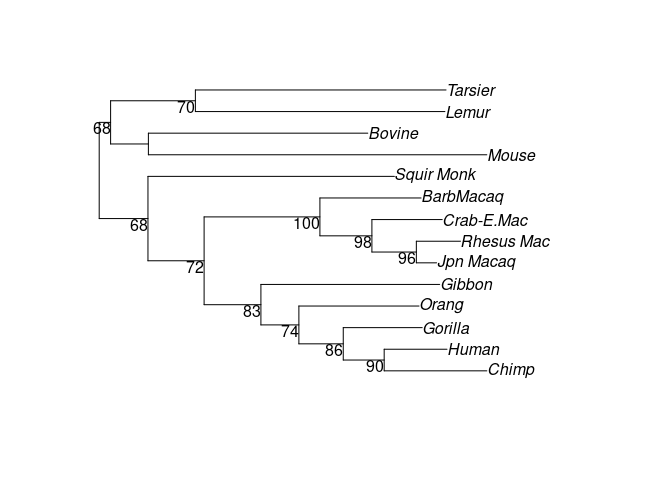Tree with bootstrap support. Unrooted tree (midpoint rooted) with bootstrap support values. cnet <- consensusNet(bs, p=0.2) plot(cnet, show.edge.label=TRUE)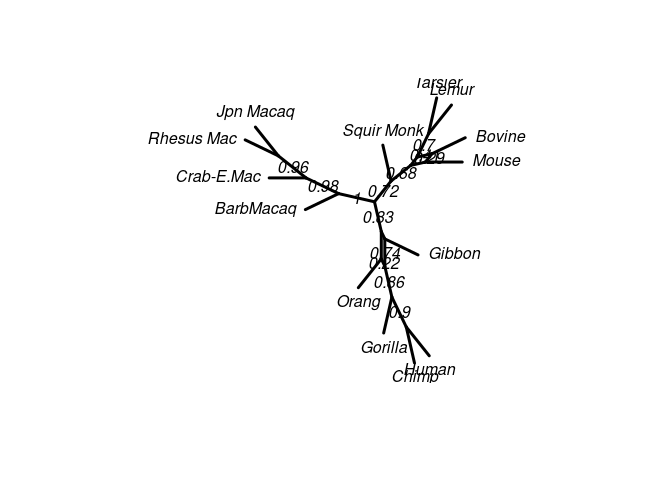ConsensusNet from the bootstrap sample. ## Molecular dating with a strict clock We implemented a strict clock as described in (Felsenstein 2004), p. ???. So far the starting tree needs to be ultrametric, so we use the UPGMA tree. When we optimise the tree with optim.pml we have to make sure set we set optRooted = TRUE! fit_strict <- pml(treeUPGMA, data=primates, k=4, bf=baseFreq(primates)) fit_strict <- optim.pml(fit_strict, model="GTR", optRooted = TRUE, rearrangement = "NNI", optGamma = TRUE, optInv = TRUE, control = pml.control(trace = 0)) plot(fit_strict)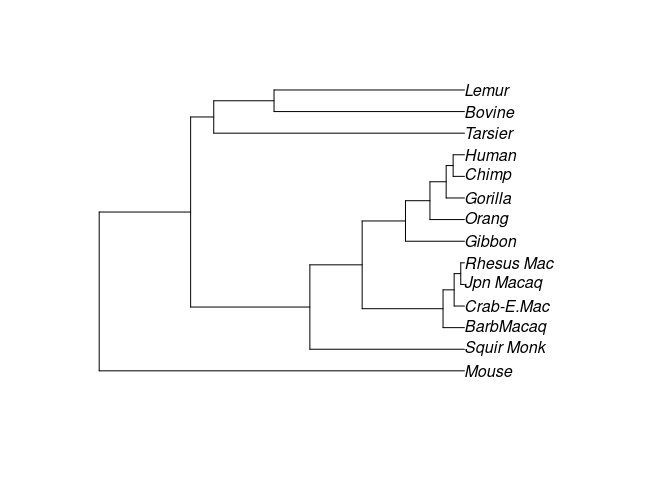Several analyses, e.g.bootstrap and modelTest, can be computationally demanding, but as nowadays most computers have several cores one can distribute the computations using the parallel package. However it is only possible to use this approach if R is running from command line (“X11”), but not using a GUI (for example “Aqua” on Macs) and unfortunately the parallel package does not work at all under Windows. # Appendix ## Standard scripts for nucleotide analysis Here we provide two standard scripts which can be adapted for the most common tasks. Most likely the arguments for read.phyDat have to be adapted to accommodate your file format. Both scripts assume that the parallel package works on your platform, see comments above. library(phangorn) file <- "myfile" dat <- read.phyDat(file) dm <- dist.ml(dat, "F81") tree <- NJ(dm) # as alternative for a starting tree: tree <- pratchet(dat) # parsimony tree tree <- nnls.phylo(tree, dm) # need edge weights # 1. alternative: quick and dirty: GTR + G fitStart <- pml(tree, dat, k=4) fit <- optim.pml(fitStart, model="GTR", optGamma=TRUE, rearrangement="stochastic") # 2. alternative: prepare with modelTest mt <- modelTest(dat, tree=tree, multicore=TRUE) mt[order(mt$AICc),]
# choose best model from the table according to AICc
bestmodel <- mt$Model[which.min(mt$AICc)]

env <- attr(mt, "env")
fitStart <- eval(get("GTR+G+I", env), env)

# or let R search the table
fitStart <- eval(get(bestmodel, env), env)
# equivalent to:   fitStart = eval(get("GTR+G+I", env), env)
fit <- optim.pml(fitStart, rearrangement = "stochastic",
optGamma=TRUE, optInv=TRUE, model="GTR")
bs <- bootstrap.pml(fit, bs=100, optNni=TRUE, multicore=TRUE)

## Standard scripts for amino acid analysis

You can specify different several models build in which you can specify, e.g. “WAG,” “JTT,” “Dayhoff,” “LG.” Optimizing the rate matrix for amino acids is possible, but would take a long, a very long time and you will need to have a large alignment to estimate all the parameters. So make sure to set optBf=FALSE and optQ=FALSE in the function optim.pml, which is also the default.

library(phangorn)
file <- "myfile"
dat <- read.phyDat(file, type = "AA")
dm <- dist.ml(dat, model="JTT")
tree <- NJ(dm)

# parallel will only work safely from command line
# and not at all windows
(mt <- modelTest(dat, model=c("JTT", "LG", "WAG"),
multicore=TRUE))
# run all available amino acid models
(mt <- modelTest(dat, model="all", multicore=TRUE))

env <- attr(mt, "env")
fitStart <- eval(get(mt$Model[which.min(mt$BIC)], env), env)

fitNJ <- pml(tree, dat, model="JTT", k=4, inv=.2)
fit <- optim.pml(fitNJ, rearrangement = "stochastic",
optInv=TRUE, optGamma=TRUE)
fit

bs <- bootstrap.pml(fit, bs=100, optNni=TRUE, multicore=TRUE)

# Session info

## R version 4.1.0 (2021-05-18)
## Platform: x86_64-pc-linux-gnu (64-bit)
## Running under: Ubuntu 20.04.2 LTS
##
## Matrix products: default
##
## locale:
##   LC_CTYPE=de_AT.UTF-8       LC_NUMERIC=C
##   LC_TIME=de_AT.UTF-8        LC_COLLATE=C
##   LC_MONETARY=de_AT.UTF-8    LC_MESSAGES=de_AT.UTF-8
##   LC_PAPER=de_AT.UTF-8       LC_NAME=C
##  LC_MEASUREMENT=de_AT.UTF-8 LC_IDENTIFICATION=C
##
## attached base packages:
##  stats     graphics  grDevices utils     datasets  methods   base
##
## other attached packages:
##  knitr_1.33     magrittr_2.0.1 phangorn_2.7.1 ape_5.5-1
##
## loaded via a namespace (and not attached):
##   igraph_1.2.6      Rcpp_1.0.7        lattice_0.20-44   R6_2.5.0
##   quadprog_1.5-8    rlang_0.4.11      fastmatch_1.1-0   highr_0.9
##   stringr_1.4.0     tools_4.1.0       parallel_4.1.0    grid_4.1.0
##  nlme_3.1-152      xfun_0.24         jquerylib_0.1.4   htmltools_0.5.1.1
##  yaml_2.2.1        digest_0.6.27     Matrix_1.3-4      codetools_0.2-18
##  sass_0.4.0        prettydoc_0.4.1   evaluate_0.14     rmarkdown_2.9
##  stringi_1.6.2     compiler_4.1.0    bslib_0.2.5.1     jsonlite_1.7.2
##  pkgconfig_2.0.3